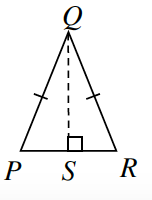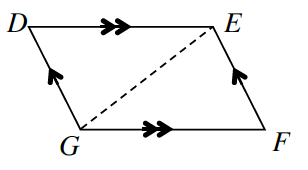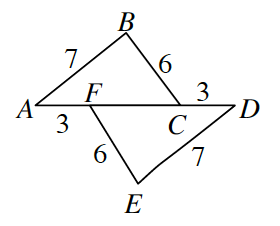### Home > INT2 > Chapter 5 > Lesson 5.1.5 > Problem5-58

5-58.

For each pair of triangles below, determine if the triangles are congruent. If the triangles are congruent,

• ​complete the correspondence statement,

• state the congruence property,

• and record any other ideas you use that make your conclusion true.

Otherwise, explain why you cannot conclude that the triangles are congruent. Note that the figures are not necessarily drawn to scale.

1. $\Delta SQP \cong \Delta$ _____$ΔSQP≅ΔSQR$ by $\text{HL}≅$

1. $\Delta EDG \cong \Delta$ _____$ΔEDG ≅ ΔGFE$ by ___ $≅$

1. $\Delta ABC \cong \Delta$ _____